# A good NIPS!

This year’s edition of NIPS was a big success. As you probably already know we had the surprise visit of Mark Zuckerberg (see here for the reason behind this visit). More interestingly (or perhaps less interestingly depending on who you are) here are some of the papers that I liked the most.

Optimization

Non-strongly-convex smooth stochastic approximation with convergence rateby Francis Bach and Eric Moulines. With noisy gradients (see this post) it is known that the best rate of convergence to minimize an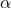-strongly convex function is of order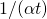while for convex functions it is of order. Unfortunately in Machine Learning applications the strong convexity parameteris often a regularization parameter that can be as small as, in which case the standard analysis using strong convexity do not yield any acceleration. In this paper Bach and Moulines show that in the case of the square loss (whose strong convexity parameter depends on the smallest non-zero eigenvalue of the covariance matrix of the covariates) one can obtain a rate of order(i.e. with no dependency on the smallest eigenvalue). The proposed algorithm is simply Stochastic Gradient Descent with a constant step-size (and averaging for the output). A more intricate algorithm is also proposed for the logistic loss.

On Decomposing the Proximal Map by Yaoliang Yu. Algorithms such as ISTA and FISTA (see this post) require to compute the proximal operatorRecall that this proximal operator arises naturally for the minimization of a function of the form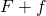, i.e. when one wants to minimize some functionwhile enforcing some of the ‘properties’ ofin the solution. For instance with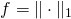one would like to output a sparse solution. Thus it is very natural to try to understand the relation between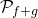and. This paper consider various properties under which one has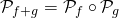.

Accelerating Stochastic Gradient Descent using Predictive Variance Reduction by Rie Johnson and Tong Zhang. This paper gives a beautiful new algorithm achieving the same performances as SDCA and SAG (see this post). The algorithm/analysis are much more intuitive than the one of SDCA and SAG. I will make a more detailed post on this paper later next year.

Accelerated Mini-Batch Stochastic Dual Coordinate Ascent by Shai Shalev-Shwartz and Tong Zhang. Both SDCA and SAG have a linear dependency on the condition number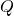. For the deterministic case Nesterov’s accelerated gradient descent attains a linear dependency on. This paper partially bridges the gap between these results and present an accelerated version of SDCA using mini batches.

Mixed Optimization for Smooth Functions by Mehrdad Mahdavi, Lijun Zhang and Rong Jin. This paper considers a new setting which seems quite natural: what if on top of noisy first order oracle one can also access a regular first order oracle? Mehrdad will do a guest post on this problem soon, but the short answer is that with only a logarithmic number of calls to the regular oracle one can attain a rate of orderfor smooth optimization (while with only the noisy oracle the rate is).

Estimation, Optimization, and Parallelism when Data is Sparse by John Duchi, Mike Jordan and Brendan McMahan. For this paper too I am hoping to have a guest post (by John Duchi this time) that would explain the new algorithm and its properties. The idea is roughly to do a gradient descent where the step-size adapts to the sparsity of the observed gradient, allowing for much faster rates in certain situations.

Bandits

Online Learning in Episodic Markovian Decision Processes by Relative Entropy Policy Search by Alexander Zimin and Gergely Neu. This paper shows that one can solve episodic loop-free MDPs by simply using a combinatorial semi-bandit strategy (see this paper by Audibert, myself and Lugosi where we solved the semi-bandit problem). I believe that this paper initiate a research direction that will be very fruitful in the future. Namely reducing (or rather reformulating) a complicated sequential decision making problem as a linear bandit (or semi-bandit). A similar approach is very popular in optimization where everyone knows that one should try very hard to formulate the problem of interest as a convex program. On the other hand such an approach in online learning/sequential decision making has not been recognized yet. I believe that at the moment the most promising direction is to try to formulate the problem as a linear bandit as it is both an extremely general problem but also one for which we have seemingly canonical algorithms. A related paper is Online Learning in Markov Decision Processes with Adversarially Chosen Transition Probability Distributions by Abbasi-Yadkori, Bartlett, Kanade, Seldin and Szepesvari.

Two-Target Algorithms for Infinite-Armed Bandits with Bernoulli Rewards by Thomas Bonald and Alexandre Proutiere. This paper considers the famous Bayesian setting of Berry, Chen, Zame, Heath and Shepp where one has a countable set of arms with Bernoulli distributions and means drawn uniformly on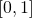. This paper shows the first optimal strategy with a Bayesian regret of order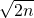. I recommend to take a look at the strategy, it is both very elegant and quite smart.

Sequential Transfer in Multi-armed Bandit with Finite Set of Models by Mohammad Azar, Alessandro Lazaric and Emma Brunskill. This paper considers an elegant and simple model for transfer learning in multi-armed bandit problems. To put it simply the setting is the one of a Bayesian multi-armed bandit where the underlying parameter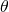is replaced by a new fresh independent sample verysteps. If the prior is known then this problem is fairly straightforward (given our current knowledge of the multi-armed bandit). The issue is what to do when the prior is unknown. The paper proposes an interesting strategy based on learning latent variable models via the method of moments (see this paper by Anandkumar, Ge, Hsu, Kakade, and Telgarsky for instance). While this gives non-trivial results I believe that much more can be said by fully embracing the sequential nature of the problem rather than trying to blend together a batch method with standard bandit strategies. I suspect that the technical difficulties to obtain an optimal strategy for this setting will be tremendous (which is quite exciting!).

Eluder Dimension and the Sample Complexity of Optimistic Exploration by Daniel Russo and Benjamin Van Roy; Prior-free and prior-dependent regret bounds for Thompson Sampling by myself and Che-Yu Liu. These two papers ask the same question (with the latter paper being inspired by the former): we know that Thompson Sampling can be as good as UCB when initialized with a well-chosen priors, but can we show that it has a significant advantage in the cases where the prior is actually informative? The first paper addresses this question in the context of linear bandits (which is, as pointed out above, the most fundamental bandit problem), while the second paper considers a much more restricted class of problems but for which surprisingly strong results can be obtained.

Online Learning

Dimension-Free Exponentiated Gradient by Francesco Orabona. This paper introduces a new regularizer for Mirror Descent and shows that it adapts automatically to the norm of the unknown comparator. In my opinion we know very few interesting regularizers for MD (especially in the full-information setting) and any non-trivial addition to this set seems difficult. This paper manages to do just that. It would be interesting to see if this gives new insights for bandit regularizers.

Minimax Optimal Algorithms for Unconstrained Linear Optimization by Brendan McMahan and Jacob Abernethy. My advice for this paper is to look at Section 3.2 which gives one very concrete and very cool application of the method they develop.

Other

I’m running out of stamina so I will now just list the other papers that I found very interesting.

Density estimation from unweighted k-nearest neighbor graphs: a roadmap by von Luxburg and Alamgir.

Stochastic blockmodel approximation of a graphon: Theory and consistent estimation by Airoldi, Costa and Chan.

Near-Optimal Entrywise Sampling for Data Matrices by Achlioptas, Karnin and Liberty.

Near-optimal Anomaly Detection in Graphs using Lovasz Extended Scan Statistic by Sharpnack, Krishnamurthy and Singh.

Estimating the Unseen: Improved Estimators for Entropy and other Properties by Valiant and Valiant.

Information-theoretic lower bounds for distributed statistical estimation with communication constraints by Zhang, Duchi, Jordan and Wainwright.

This entry was posted in Conference/workshop. Bookmark the permalink.

## 3 Responses to "A good NIPS!"

•